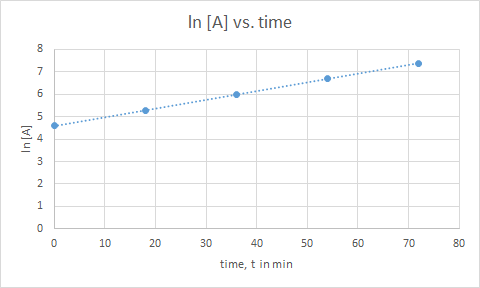# Problem: Suppose that you place exactly 100 bacteria into a flask containing nutrients for the bacteria and that you find the following data at 37 °C:       t (min)                Number of bacteria                  0                                 100        18.0                             200        36.0                             400        54.0                             800        72.0                             1600What is the order of the rate of production of the bacteria? a) zero order b) first order c) second order How many bacteria will be present after 162 min? What is the rate constant for the process?

###### FREE Expert Solution

If plot is a straight line for:

• [A] vs. t → zero order
• ln [A] vs. t → first order
• 1/[A] vs. t second order###### Problem Details

Suppose that you place exactly 100 bacteria into a flask containing nutrients for the bacteria and that you find the following data at 37 °C:

t (min)                Number of bacteria

0                                 100

18.0                             200

36.0                             400

54.0                             800

72.0                             1600

What is the order of the rate of production of the bacteria?

a) zero order

b) first order

c) second order

How many bacteria will be present after 162 min?

What is the rate constant for the process?